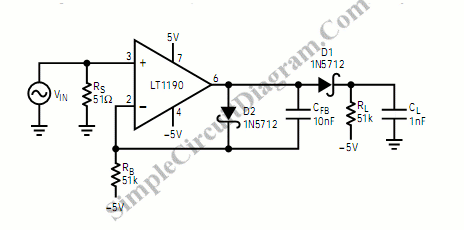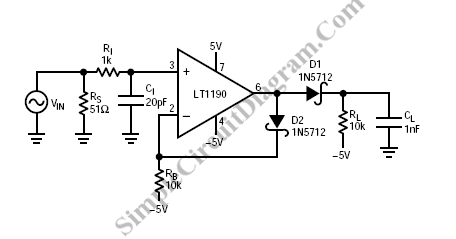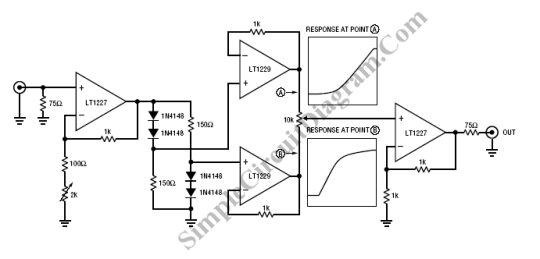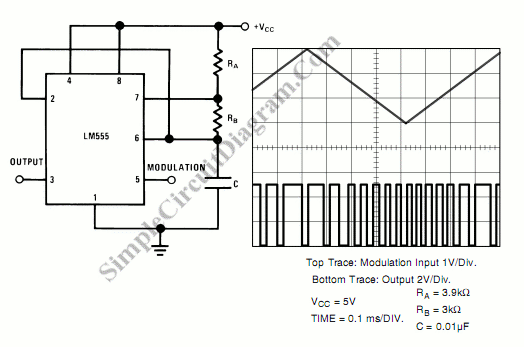## Open Loop Fast Peak DetectorIn this peak detector circuit, D1 is the detector diode and D2 is a level shifting or compensating diode. A load resistor RL is connected to – 5V and an identical bias resistor, RB, is used to bias the compensating diode.  This  equal value resistors RL and RB make sure that the diode drops are equal. High values of RB […]

## Fast Pulse DetectorA schematic diagram of a fast pulse detector is show in the figure below.  Error detection rate under 10% can be expected for 60 ns pulse. To get error free performance, it is recommended to use this circuit for detecting 100 ns or wider pulse. [Schematic diagram source: Linear Technology Application Notes]

## Gamma Correction CircuitGamma correction need to be applied in video processing since the transfer function of video acquisition and video display is non linear.  Here is the schematic diagram of a gamma circuit: If gamma value equal to 1, the the  transfer function is linear. Typical CRT usually have  gamma factor from about 2.0 to 2.4 in their transfer function.  The effect […]